# Math Practice Topic: Quadratic Graph Zeros

 Description: Find solution(s) to a quadratic equation, given the graph. Adaptive Learning Progression: The equations become harder to factor. Start using MathScore for free

## Sample Levels (out of 5)

Find the zeros (roots) of the following functions. Whenever there are multiple answers, separate them with commas. Example: 1,3

 1 y = x 2 - 12x - 13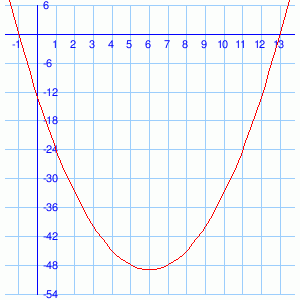x =

Find the zeros (roots) of the following functions. Whenever there are multiple answers, separate them with commas. Example: 1,3

 1 y = x 2 + 15x + 14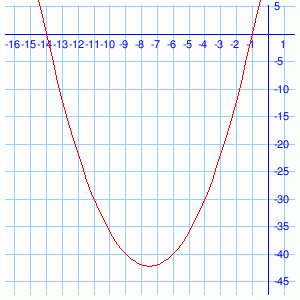x = 2 y = - 3x 2 - 42x - 147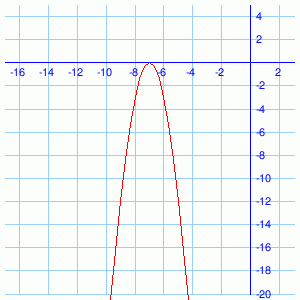x = 3 y = x 2 + 21x + 38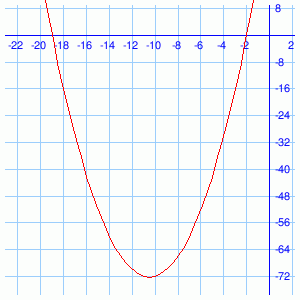x = 4 y = -x 2 - 6x - 9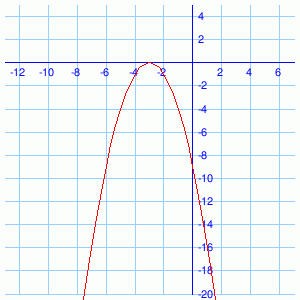x =